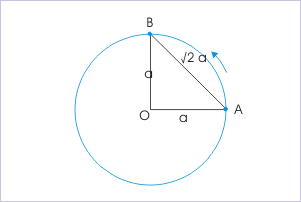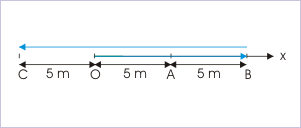# 1.12 Displacement  (Page 5/5)

 Page 5 / 5

Therefore, what we mean by the inequality emphasized earlier is that change in the magnitude of position vector, "r”, is not equal to the magnitude of change in the position vector. In order to appreciate the point, we can consider the case of two dimensional circular motion. Let us consider the motion from point "A" to "B" along a circle of radius "a", as shown in the figure.A particle transverses a quarter of circle from A to B.

Since radius of the circle remains same, the change in the magnitude of position vector,"r", is zero during the motion. Hence,

$\begin{array}{l}\Delta r=0\end{array}$

However, the magnitude of displacement during the motion is :

$\begin{array}{l}\mathrm{AB}=\surd \left({a}^{2}+{a}^{2}\right)=\surd 2a\end{array}$

Hence, the magnitude of change in the position vector is :

$\begin{array}{l}|\Delta \mathbf{r}|=\mathrm{AB}=\surd 2a\end{array}$

Clearly,

$\begin{array}{l}\Delta r\ne |\Delta \mathbf{r}|\end{array}$

Further, | r | represents the magnitude of position vector and is equal to “r” by conventional meaning. Hence,

$\begin{array}{l}\Delta r=\Delta |\mathbf{r}|\end{array}$

Therefore,

$\begin{array}{l}\Delta |\mathbf{r}|\ne |\Delta \mathbf{r}|\end{array}$

This completes the discussion on similarities and differences among the four symbols. But, the question remains why there are differences in the first place. The answer lies in the fact that position vector is a vector quantity with directional property. It means that it can change in either of the following manner :

1. change in magnitude
2. change in direction
3. change in both magnitude and direction

Thus we see that it is entirely possible, as in the case of circular motion, that change in position vector is attributed to the change in direction alone (not the magnitude). In that case, Δr and |Δ r | are not same. We can see that such difference in meaning arises due to the consideration of direction. Will this difference persist even in one dimensional motion?

In one dimensional motion, representations of change in position vector and displacement are done with equivalent scalar system. Let us examine the meaning of equivalent scalar terms. Here, the change in the magnitude of position vector "r" is equivalent to the change in the magnitude of position vector, represented by scalar equivalent "|x|". Also, the magnitude of change in the position vector “ r ” is equivalent to the magnitude of change in the position vector, "x" (note that signed scalar "x" denotes position vector).

Let us consider the case of a rectilinear motion (motion along a straight line) taking place from from A to B to C. The magnitude of change in the position vector, “x”, considering “O” as the origin is :A particle moves along a straight line.

$\begin{array}{l}|\Delta x|=|{x}_{2}-{x}_{1}|=|-5-5|=10\phantom{\rule{2pt}{0ex}}m\end{array}$

Now, the change in the magnitude of position vector, “|x|”, is :

$\begin{array}{l}\Delta |x|=|{x}_{2}|-|{x}_{1}|=5-5=0\end{array}$

Thus we see that difference in two terms exist even in one dimensional motion. This is expected also as one dimensional motion can involve reversal of direction as in the case considered above. Hence,

$\begin{array}{l}\Delta |x|\ne |\Delta x|\end{array}$

Let us now consider unidirectional one dimensional motion like uniform motion in which velocity is constant and particle moves in only one direction. In this case, this difference disappears and

$\begin{array}{l}\Delta |x|=|\Delta x|\end{array}$

The discussion here on this subtle difference is very important as this becomes an important consideration subsequently with velocity and acceleration as well, which are defined in terms of position vector.

## Example

Problem : The displacement (x) of a particle is given by :

$\begin{array}{l}x=A\mathrm{sin}\left(\omega t+\theta \right)\end{array}$

At what time from the start of motion is the displacement maximum?

Solution : The displacement (x) depends on the value of sine function. It will be maximum for maximum value of sin(wt + θ). The maximum value of sine function is 1. Hence,

$\begin{array}{l}\mathrm{sin}\left(\omega t+\theta \right)=1=\mathrm{sin}\left(\frac{\pi }{2}\right)\\ ⇒\omega t+\theta =\pi 2\\ ⇒t=\frac{\pi }{2\omega }-\frac{\theta }{\omega }\end{array}$

The motion is oscillating as expression for displacement is sinusoidal. The particle will attain maximum displacement at regular intervals.

#### Questions & Answers

What are the system of units
Jonah Reply
A stone propelled from a catapult with a speed of 50ms-1 attains a height of 100m. Calculate the time of flight, calculate the angle of projection, calculate the range attained
Samson Reply
58asagravitasnal firce
Amar
water boil at 100 and why
isaac Reply
what is upper limit of speed
Riya Reply
what temperature is 0 k
Riya
0k is the lower limit of the themordynamic scale which is equalt to -273 In celcius scale
Mustapha
How MKS system is the subset of SI system?
Clash Reply
which colour has the shortest wavelength in the white light spectrum
Mustapha Reply
how do we add
Jennifer Reply
if x=a-b, a=5.8cm b=3.22 cm find percentage error in x
Abhyanshu Reply
x=5.8-3.22 x=2.58
sajjad
what is the definition of resolution of forces
Atinuke Reply
what is energy?
James Reply
Ability of doing work is called energy energy neither be create nor destryoed but change in one form to an other form
Abdul
motion
Mustapha
highlights of atomic physics
Benjamin
can anyone tell who founded equations of motion !?
Ztechy Reply
n=a+b/T² find the linear express
Donsmart Reply
أوك
عباس
Quiklyyy
Sultan Reply
Moment of inertia of a bar in terms of perpendicular axis theorem
Sultan Reply
How should i know when to add/subtract the velocities and when to use the Pythagoras theorem?
Yara Reply

### Read also:

#### Get the best Physics for k-12 course in your pocket!

Source:  OpenStax, Physics for k-12. OpenStax CNX. Sep 07, 2009 Download for free at http://cnx.org/content/col10322/1.175
Google Play and the Google Play logo are trademarks of Google Inc.

Notification Switch

Would you like to follow the 'Physics for k-12' conversation and receive update notifications?ByBy